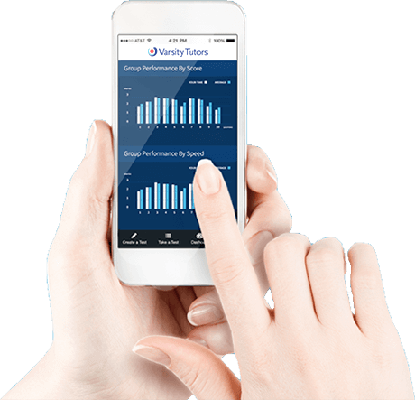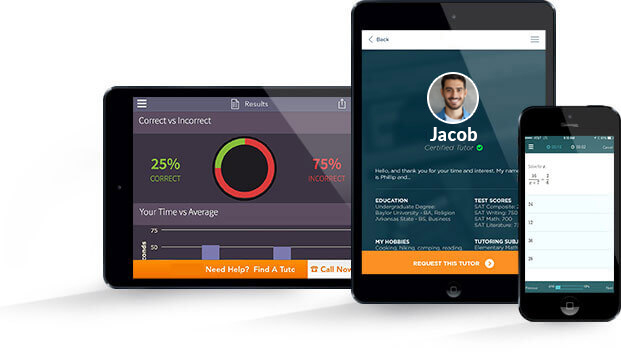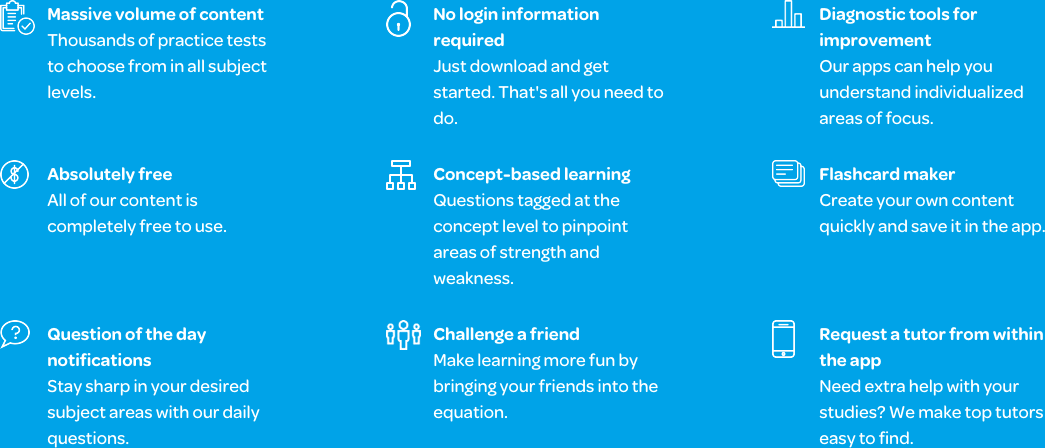# Varsity Tutors SAT II Math mobile app### The Varsity Tutors SAT II Math Mobile App

The SAT Subject Test in Math 1 is a great way for you to demonstrate your interest and proficiency in math skills to prospective colleges. Formerly known as the SAT II Math 1 test, it covers algebra, functions, and geometry. It may also contain questions about data analysis, statistics, or probability. The test may briefly address trigonometry as well. With the free Varsity Tutors SAT Math 1 app for iPhone, iPad, and Android devices, you can prepare to get the best score possible in order to impress prospective universities and colleges.

The SAT Math 1 Subject Test study app is a fully customizable way to study for the test. You can begin by taking one of the full-length, timed practice tests to see where your skills are. These tests provide comprehensive results that help you discover which areas you already know well, and the concepts on which you may need some review. The results page also includes full explanations of the correct answers and links to relevant concept review opportunities.

Once you know which skills you need to strengthen, you can use Learn by Concept or the flashcards to study those areas. Looking for a little work on matrices? Tap to review that subject, practice with flashcards, or take a concept-specific practice test to work with the kind of problems you will be given on the real SAT Math 1 Subject Test. These practice tests also come with detailed explanations of the correct answers. You can even use the Flashcard Maker to create your own flashcards, using not only words, but images and sound as well.

In the data analysis and statistics section of the test, you will need to know how to work with mean, median, mode, probability, and range. The functions and graphs section encompasses graphing functions such as linear and quadratic functions. It also includes properties of functions and graphs such as maximum and minimum, and domain and range. You’ll need to be able to solve many types of functions, including some you’ll interpret from word problems.

The geometry covered on the SAT Math 1 Subject Test includes coordinate, 2-dimensional, and 3-dimensional geometry. In coordinate geometry, you will need to work with points, lines, and rays, using the distance and midpoint formulas to measure lines. In 2-dimension geometry, you’ll work with shapes such as circles, hexagons, triangles, and quadrilaterals, using formulas to find such things as perimeter and area. Finally, in 3-dimensional geometry, you will work with shapes like cones, spheres, and pyramids, where you will use formulas to find surface area and volume.

Mathematical relationships are also important to know; absolute value, properties and identities, and other concepts will be tested. Number theory and single-variable algebra are covered as well.

Using the Varsity Tutors SAT Math 1 Subject Test app is an easy, free way to review. It was developed to mimic the structure of the actual SAT Subject Test you will be taking to best prepare you for the big day. Download the app from iTunes or the Google Play Store.Your knowledge of algebra and geometry are assessed with the SAT Subject Test in Math Level I, previously known as the SAT II Math I. The SAT Subject Tests are used to show your knowledge and skill level. A good score looks nice on your college application and positively compares your abilities with other students who apply to the same school. The exam is meant to assess what you know after three years of high school math. If you’re planning to study a field in technology, science, economics, or engineering, it can be helpful during the admissions process.

The SAT Math I has 50 questions, which you have 60 minutes to answer. Each question is in multiple-choice format, and the test is scored on a 200-800 point system. Each correct answer amounts to one point. If a question is answered incorrectly, then you will lose one-quarter of a point, and get no points if a question is left blank. Once a raw score is tallied, it’s then converted into a scaled score. The primary topics are numbers and operations; algebra and functions; geometry and measurement; and data analysis, statistics, and probability. You’ll be asked to work on problems involving operations, complex numbers, ratio and proportion, and counting. Matrices and sequences may be included in problems as well.

During the test, you will be allowed to use a calculator. The test policy determines the exact types you’ll be able to use, as determined by The College Board. You can only use battery operated calculators and those that don’t have a QWERTY keyboard, make noise, or use paper tape. The device cannot be part of a cell phone or PDA, nor can they require a stylus to operate. Read up on the rules beforehand so there are no conflicts during the exam.

With the statistics and probability questions you’ll be facing on the exam, you will have to be familiar with graphing and plotting, mean, mode, median, and range. These may seem like tough concepts, but they factor into many aspects of the real world, including population analysis, economics, and marketing. The questions may also include familiar concepts from high school algebra. Each expression you have learned has symbols that represent numbers and mathematical operations, and is manipulated using exponents, for example. In its most basic form, an equation represents an equality between two expressions. The values on either side of the equation are the solutions. An equation with one variable gives you a number. If there are two variables, you can arrive at a set of ordered pairs that can be plotted on a coordinate plane.

As in class, the algebra on the test will prompt you to interpret the structure of expressions and write these in equivalent forms in order to solve specific problems. You also have to do arithmetic on polynomials on the SAT Subject Test in Math Level I. The relationship between zeros and factors of polynomials is essential to understand, as are polynomial identities and rational functions. You should have the ability to create equations based on the numbers or relationships involved, and to reason with equations and inequalities. The test includes solving systems of equations and graphically representing these solutions.

The test will also focus on Euclidean geometry. It includes concepts such as congruence, which is applied to geometric theorems. Right triangles are an important part of geometry, and they serve as an introduction to trigonometry. Here is where the Pythagorean Theorem, which identifies the relationship between the hypotenuse (long side) and other two sides of a right triangle, applies. Various theorems on circles are covered by the test, and you should know how to find arc lengths and the area of certain parts of a circle. You’ll also have to work with geometric properties, and use equations to express these. Using mathematical equations, you can translate between a representation of an object or shape and the geometric description. You also use algebra to prove geometric theorems; coordinates are often integral to this process. Volume formulas and dimensions link the mathematical and visual elements of geometry.

Algebra and geometry are linked through analytic geometry. It expands your problem-solving abilities, and is something that needs to be mastered for the SAT Math I. This topic is required understanding for analyzing geometric points in two dimensions, and ultimately in three. From modeling a problem to proving a theorem, you need a breadth of knowledge to help answer the tougher questions on the test. These are sure to include problems involving lines, circles, and parabolas. More advanced operations, including finding the volume and surface areas of three-dimensional solids, such as cylinders, cones, and spheres, will be required. And don’t forget to brush up on trigonometry and the characteristics of different kinds of triangles.

People often have a choice between the Level I or Level II tests. The SAT Subject Test in Math Level I includes working with complex numbers; algebraic equations and functions; Euclidean geometry; and working with mean, median, range, and mode. It has data interpretation and probability questions. By contrast, the Level II test asks about exponential and quadratic regression and some other more advanced topics. Not everyone is as advanced in math at this stage, but that doesn’t mean you can’t demonstrate valuable skills to improve on your college application. Plus, administrators will want to know exactly how proficient you are in the subject.

The SAT Math Level I is just one of several subjects you can be tested on through the College Board’s SAT Subject Test program. If you’ve decided to take the math test, you have likely chosen an academic path that involves knowing and mastering certain concepts in math. The test may be required by your high school or the colleges or universities you apply to. Algebra and geometry may have been your strong points throughout high school. If that’s the case, you can potentially make yourself a more suitable candidate for your desired college program with a good score on the SAT Math I.